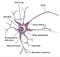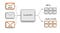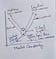# Deep Learning — a foundation

The whole idea behind deep learning is to have the computers artificially mimic biological natural intelligence, so let’s build a general understanding of how biological neurons works.Figure 1: Biological Neuron
• This is biological neurons, the main thing to note here is various parts are connected to nucleus and the axon takes the part output from nucleus.

.

.

.

.

# Evaluating Performance— Regression

Whatever we do, we need evaluation to let us know right or wrong.

I believe you all know what is Regression task. For example

• Attempting to predict the price of a house given its feature is a regression task
• Attempting to predict the country a house is in given its feature would be a classification task.

The most common evaluation metrics for regression:

→ Mean Absolute Error (MAE).

→ Mean Squared Error (MSE).

→ Root Mean Squared Error (RMSE).

## Mean Absolute Error:

• Mean Absolute Error is calculated by taking the mean of absolute value of the difference between actual and predicted value.

# Logistic RegressionFigure 1: Classification Algorithm

Linear Regression works for continuous variable prediction. EX: Prediction of percentage mark scored by the student.

Logistic Regression works for discrete variable prediction. EX: Predicting Pass or Fail of the Student.

# Regularization- Lasso, Ridge, Elastic Net.

Why do we need regularization methods? Answer: If the Linear Regression model leads to overfitting, we apply regularization techniques to avoid overfitting issue.

Before getting into regularization techniques, we can understand about overfitting and underfitting.

## Bias and Variance - Trade-off:

Bias - Error between average model predictions and ground truth.

Variance -Average variability in the model prediction for the given dataset.Fig 1 : Bias-Variance Trade-off

# Linear Regression — Icebreaker to Machine Learning Algorithms.

Linear Regression is the first step to climb the ladder of machine learning algorithm. Linear Regression comes under supervised learning where we have to train the Linear Regression model to predict data.

## Introduction:

• Francis Galton was studying the relationship between parents and children in 1800's.
• He stated there is correlation and causation between parents and children.

The Goal is to find the best fit line by minimizing the vertical distance, i.e. The error between predicted and actual value.

Linear regression is used for finding linear relationship between target and one or more predictors. …## Rishi Kumar

I'm a passionate and disciplined Data Science enthusiast.